# The value of g ( 0 ) and g ( 6 ) .### Single Variable Calculus: Concepts...

4th Edition
James Stewart
Publisher: Cengage Learning
ISBN: 9781337687805### Single Variable Calculus: Concepts...

4th Edition
James Stewart
Publisher: Cengage Learning
ISBN: 9781337687805

#### Solutions

Chapter 5.4, Problem 4E

(a)

To determine

## The value of g(0) and g(6).

Expert Solution

The value of g(0) is 0.

The value of g(6) is 0_

### Explanation of Solution

Given information:

The equation is g(x)=0xf(t)dt.

The graph is given for the integral function g(x)=0xf(t)dt.

Calculation:

Show the integral function as below.

g(x)=0xf(t)dt (1)

Here, g(x) is area under the graph of f from a to x and f(t) is function of t.

Determine g(0) using Equation (1).

Substitute 0 for x in Equation (1).

g(0)=00f(t)dt=0

Therefore, the g(0) is 0_.

Determine g(6) using given graph:

Refer the graph.

The curve is symmetrical about the point (3, 0). Hence, the area between the points 0 to 3 and 3 to 6 are equal with alternative sign.

Therefore, the function g(6) is 0.

(b)

To determine

### The value of g(x), for x=1,x=2, x=3, x=4, and x=5.

Expert Solution

The value of g(1) is 2.8.

The value of g(2) is 4.9.

The value of g(3) is 5.7.

The value of g(4) is 4.9.

The value of g(5) is 2.8.

### Explanation of Solution

Given information:

The equation is g(x)=0xf(t)dt.

The graph is given for the integral function g(x)=0xf(t)dt.

Calculation:

Draw the graph for calculation of g(1) as in Figure 1.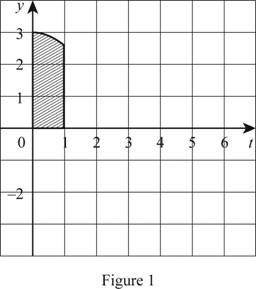Determine g(1) using Equation (1).

Substitute 1 for x in Equation (1).

g(1)=01f(t)dt (2)

Refer Figure (1).

Area of shaded rectangle is the function of t with limits 0 to 1.

Modify Equation (2).

g(1)=bh

Substitute 1 for b, 2 for h.

g(1)=bh+80%=(1)(2)+0.8=2.8

Therefore, g(1) is 2.8.

Draw the graph for calculation of g(2) as in Figure 2.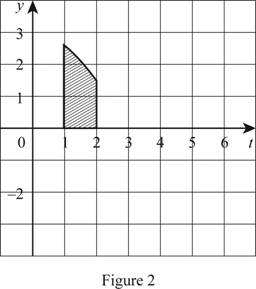Determine g(2) using Equation (1).

Substitute 2 for x in Equation (1).

g(2)=02f(t)dt=01f(t)dt+12f(t)dt=g(1)+12f(t)dt (3)

Refer Figure (2).

Area of shaded rectangle is the function of t with limits 1 to 2.

Substitute 2.8 for g(1) and bh for 12f(t)dt in Equation (3).

g(2)=g(1)+12f(t)dt=2.8+bh

Substitute 1 for b and 1 for h.

g(2)=2.8+1+0.9=4.7

Therefore, g(2) is 4.7.

Draw the graph for calculation of g(3) as in Figure 3.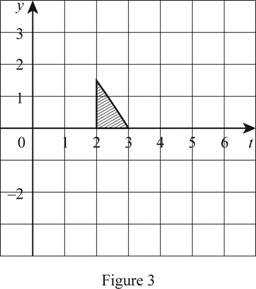Determine g(3) using Equation (1).

Substitute 3 for x in Equation (1).

g(3)=03f(t)dt=01f(t)dt+12f(t)dt+23f(t)dt=g(2)+23f(t)dt (4)

Refer Figure 3.

Area of shaded triangle is the function of t with limits 2 to 3.

Substitute 4.7 for g(2) and bh for 32f(t)dt in Equation (4).

g(3)=g(2)+32f(t)dt=4.7+12bh

Substitute 1 for b and 1.2 for h.

g(3)=g(2)+32f(t)dt=4.7+12(1)(1.2)=5.3

Therefore, g(3) is 5.3.

Draw the graph for calculation of g(4) as in Figure (4).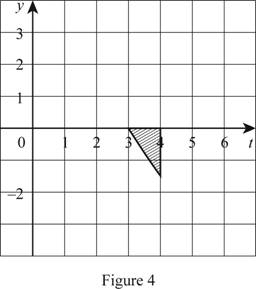Determine g(4) using Equation (1).

Substitute 4 for x in Equation (1).

g(4)=04f(t)dt=01f(t)dt+12f(t)dt+23f(t)dt+34f(t)dt=g(3)+34f(t)dt (5)

Refer Figure 4.

Area of shaded triangle is the function of t with limits 3 to 4.

Substitute 5.3 for g(3) and (12bh) for 34f(t)dt in Equation (5).

g(4)=g(3)34f(t)dt=5.312bh

Substitute 1 for b and 1 for h.

g(4)=5.312bh=5.312(1)(1.2)=4.7

Therefore, g(3) is 5.3.

Draw the graph for calculation of g(5) as in Figure 5.Determine g(5) using Equation (1).

Substitute 5 for x in Equation (1).

g(5)=05f(t)dt=01f(t)dt+12f(t)dt+23f(t)dt+34f(t)dt+45f(t)dt=g(4)+45f(t)dt (6)

Refer to Figure 5.

Area of shaded portion is the function of t with limits 4 to 5.

Substitute 4.7 for g(4) and bh for 45f(t)dt in Equation (6).

g(5)=g(4)45f(t)dt=4.7bh

Subtract 90% of third unit square.

Substitute 1 for, 1 for h.

g(5)=4.7bh=4.7(1)(1)0.9=2.8

(c)

To determine

### The interval when g is increasing.

Expert Solution

The function g is increasing at the interval (0,3)_.

### Explanation of Solution

Given information:

The equation is g(x)=0xf(t)dt.

The graph is given for the integral function g(x)=0xf(t)dt.

Calculation:

Refer to Part (a).

The value of g is increasing from the interval 0 to 3.

Therefore, the function g is increasing at the interval (0,3)_.

(d)

To determine

### The location of maximum value of g.

Expert Solution

The maximum value of g lies at x=3_.

### Explanation of Solution

Given information:

The equation is g(x)=0xf(t)dt.

The graph is given for the integral function g(x)=0xf(t)dt.

Calculation:

Refer part (a) calculation

Maximum value of g lies at x=3

Therefore, the maximum value of g lies at x=3.

(e)

To determine

Expert Solution

### Explanation of Solution

Plot the graph for function f using the calculated values of 0, 2.8, 4.7, 5.3 and 4.7 for the functions g(1), g(2), g(3), g(4), and g(5) respectively.

Show the graph for function f as in Figure 6.(f)

To determine

Expert Solution

### Explanation of Solution

Show the graph for function g(x) as in Figure 7.

Draw the graph g(x) as shown in Figure 7.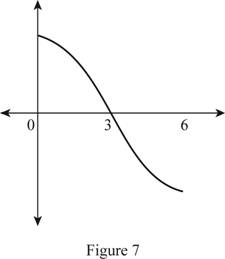### Have a homework question?

Subscribe to bartleby learn! Ask subject matter experts 30 homework questions each month. Plus, you’ll have access to millions of step-by-step textbook answers!